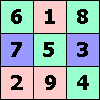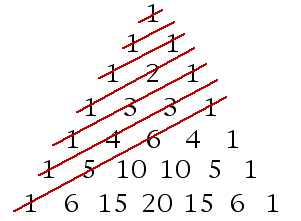ONE TO NINE by Andrew HodgesPage 181:

It is easy to see that 5! = 5 × 4 × 3 × 2 × 1 = 120 has a 0 at the end, and that further multiplications by 6, 7, 8... will always leave a final 0.

The more difficult question is how many zeroes appear. The EASY question has drawn attention to the fact that the first 0 appears not for 10!, but for 5!. This is because a 0 at the end of a number means that the number can be divided by 10, i.e by the prime factors 5 and by 2. Now, there are many factors of 2 going into n!, far more than there are factors of 5. So we need not worry about the factors of 2; knowing the number of zeroes is equivalent to counting the number of factors of 5.

At first sight, going through the numbers from 1 to 100 will hit 20 numbers which contribute a factor of 5 (i.e. 5, 10, 15, 20... 95, 100).

However, 100 itself will clearly contribute two more zeroes, so this cannot be the whole story. Looking at this in terms of prime factors, the point is that 100 has two factors of 5. Are there any other numbers with two factors of 5? Yes, 25, 50 and 75. So the total number of zeroes in 100! is 24.

For 1000! the count can follow the same principle. Going from 1 to 1000 there are 200 multiples of 5, 40 multiples of 25, 8 multiples of 125, and 1 multiple of 625. These contribute 200 + 40 + 8 + 1 = 249 zeroes.

10000! has 2499 zeroes. Why is this almost exactly 1/4 of 10000? Hint: you can use the formula for geometric progressions from Chapter 9.

Page 182:

On-line 'roulette' playing, enormously promoted by spam emails, has re-cycled a classic 'betting strategy' based on increasing the stake every time you lose. The guide at www.roulette-cheat-guide.com, for instance, urges you to play this strategy. This particular example involves betting on events with a probability of 1/3, and increasing the stake by 50% each time a loss is made, but the general principle and the fallacy behind it can be illustrated by the simpler example of betting on coin-tossing.

Assume the game is played with a fair coin, and that I win with a head (H) and get back double my stake; with a tail (T) I lose my stake. Then the strategy is to start with a bet of £1. If I win, I gain £1. If I lose on the first toss then I bet £2. If I win on the second toss then my overall gain is still £1. If I lose, then I bet £4 on a third toss. If that comes up heads then my overall gain is still £1 (£8 &minus £1 &minus £2 &minus £4). If I lose, I go on doubling, and whenever a head comes up, my overall gain is £1, and then I start all over again.

Can't lose? The snag is that my resources are finite. Suppose I have only £1023 available. Then if the sequence T T T T T T T T T T occurs, I lose it all. This sequence will come up on average 1 time in 1024. On average, the loss of £1023 once in 1024 games will exactly balance the gains of £1 in the other 1023 games.

The strategy prescribed in www.roulette-cheat-guide.com is just like this: your entire pot of \$200 will be lost in 1 out of about 87 sequences, exactly cancelling out the (average) gain of about \$2.34 that arises from the other 86.

Note: The stakes specified in the strategy do not go up by exactly 50% but are rounded to integers: \$1, \$2, \$3, \$4, \$6, \$9, \$13, \$20, \$30, \$45, \$67. This doesn't affect the principle. There are two errors in the 'winnings' column, which in both cases actually understate them!

This means that many players of the roulette strategy will start by winning \$100, \$200, \$300 or more, and no doubt genuinely report their delight in its proved 'success'. (The chances of playing 1024 successful runs with the coin-tossing strategy, or of playing 87 runs with the roulette strategy, are both approximately the 36% that is discussed in Chapter 6.) These players' gains will, however, be balanced by the losses of those who hit the \$200 wipe-out early on and are not so delighted.

The analysis above depends on the coin-tossing or roulette simulator being genuinely random. Interestingly, www.roulette-cheat-guide.com makes the suggestion that the roulette software is actually non-random in a way that gamblers can exploit. In fact it asserts, though without any evidence, that it caters to the 'gamblers' fallacy', by making it artificially unlikely for long sequences of similar outcomes to occur. If this were really true then a gambler could indeed exploit it. But the strategy would depend entirely on this non-randomness.

Unless there is such a non-randomness to be exploited, the prospects of success grow even dimmer when we take into account the effect of the zero on the roulette wheel, which so far we have ignored. The strategy of www.roulette-cheat-guide.com is based on gambling on a 1-in-3 chance, which will pay out 3 times the stake in the event of a win. The presence of the zero means that the probability of a win is not actually 1/3 but 12/37. The effect of this is that the chance of a losing streak of 11, in which you lose your entire pot, is significantly increased. It is about one in 87, when there is no zero on the wheel, but one in 75 when there is a zero. The losses will outweigh the gains, by an average of about 40 cents a game.

Does it help to increase your limit? No, it makes it worse. If you set a limit of \$40000 then disaster strikes when there is a streak of 22 losses. Without a zero this will happen only once in 7481 games, but with a zero it will happen once in 5568 games. The net average loss per game is actually about twice as great as before.

Page 187:

There is a full description of the Enigma and its plugboard attachment on Tony Sale's Enigma pages.

In what follows it is useful to use the symbol C(n, r) to mean the number of ways of choosing r things out of n, i.e. the number n!/(r! × (n − r)!).

We want to know the number of ways of putting 10 connecting wires into the plugboard, which is the same as choosing 10 pairs out of the 26 letters.

One way of doing this is as follows: suppose that we had ten differently coloured connecting wires: red, blue, green, etc etc.

Then there are C(26,2) ways of choosing a pair for the red wire. For each of these there are C(24,2) ways of choosing a pair for the blue wire, and so on, giving the product

C(26,2) × C(24,2) × C(22,2) × ... × C(8,2)

This can be simplified, with many factors cancelling, to

26! / (6! 210)

But in the actual Enigma the wires are not coloured. This means we must divide by the number of ways of permuting the 10 coloured wires, i.e. divide by a further factor of 10!. This gives the answer:

26! / (6! 10! 210) = 150,738,274,937,250.

More abstractly: the number of ways of choosing m pairs out of n objects is:

n! /((n-2m)! m! 2m)

If you want to convince yourself of this formula you might like to check that there are:

• 3 different ways of putting 2 pairs of wire into 4 plugboard sockets
• 15 different ways of putting 3 pairs of wire into 6 plugboard sockets.

From this formula we can find out something which often surprises people, which is that the number of possible plugboard pairings is greatest for 11 pairs, and then decreases:

1 pair: 325
2 pairs: 44,850
3 pairs: 3,453,450
4 pairs: 164,038,875
5 pairs: 5,019,589,575
6 pairs: 100,391,791,500
7 pairs: 1,305,093,289,500
8 pairs: 10,767,019,638,375
9 pairs: 58,835,098,191,875
10 pairs: 150,738,274,937,250
11 pairs: 205,552,193,096,250
12 pairs: 102,776,096,548,125
13 pairs: 7,905,853,580,625

If you don't believe this can happen, make a list of the number of ways you can put 2 pieces of wire into 6 plugboard sockets. There are 45 ways, three times as many as you get with 3 wires. (Hint: imagine pulling out one of the three wires.)Page 197:

There cannot be a value for (−1)! consistent with the definition of the ! symbol. It would be necessary to have 0! = 0 × (− 1)!. But 0! = 1, so this is impossible.

Page 198:

The formula works out as follows:

n=2: π/4 ≈ 0.7854
n=3: π/6 ≈ 0.5236
n = 4: π2/32 ≈ 0.3084
n = 5: π2/60 ≈ 0.1645
n = 6: π3/384 ≈ 0.0807
n = 7: π3/840 ≈ 0.0369
n = 8: π4/6144 ≈ 0.0159
n = 9: π4/15120 ≈ 0.0064
n = 10: π5/122880 ≈ 0.0025

The values must decrease as n increases, because the more squares are added together, the less likely it is that the sum will still be less than 1.

In the case n = 2, the formula can be interpreted as giving the probability that a point in a square, picked randomly, should be inside the circle. For n = 3, you can look at that picture as representing a sphere lying inside a cube, and the formula as giving the ratio of the volumes. For n = 4, 5, 6... the formula goes on to give the corresponding ratio of hyperspheres to hypercubes.

Page 200:

The rows of the Pascal triangle sum to successive powers of 2. To see why this is, think of each entry in a row as being copied twice over into the row below: once to the left and once to the right. Therefore the whole row is copied twice over, and so the row below has twice the sum.These diagonals sum to Fibonacci numbers.

Pages 202-4: Investigation of the Euler numbers 1, 1, 5, 61, 1385, 50521... shows their connections not only with the geometry of the circle and with alternating permutations, but with quantum mechanics. My colleague Sukumar and I have shown a new way to classify permutations so as to bring out this unsuspected structure. There is a description of our observations on a page of this website.

Page 212: Paper in The Lancet on mortality in Iraq.